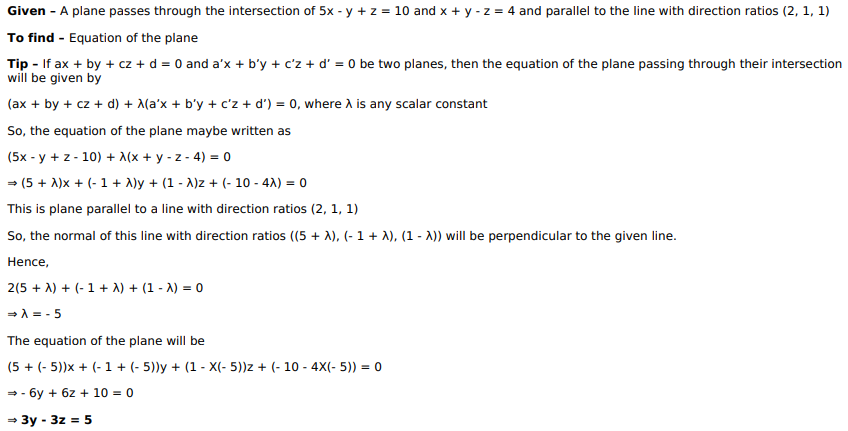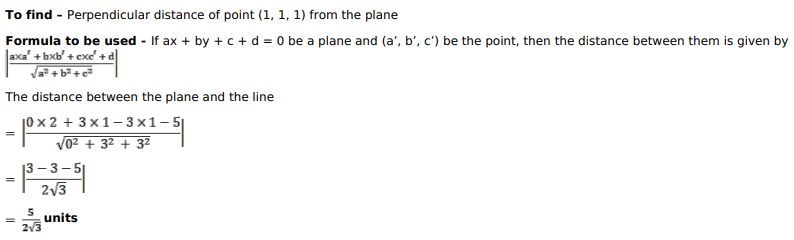# Find the equation of the plane passing through the intersectionQuestion:

Find the equation of the plane passing through the intersection of the planes5x $-y+z=10$ and $x+y-z=4$ and parallel to the line with direction ratios2, 1,1 . Find also the perpendicular distance of $(1,1,1)$ from this plane.

Solution: